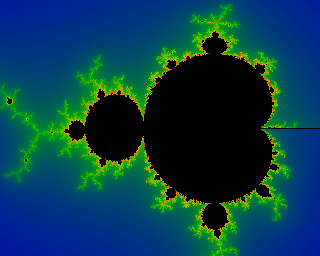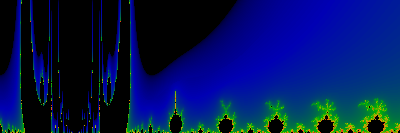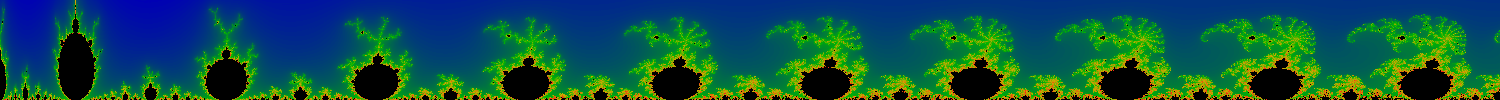Self Similar?

Fractals are by definition self-similar, and the M-set is obviously self-similar by visual examination. But are there any exact expressions? Is there any chance that the non-roundness of the buds is explainable through some psuedo-conformal distortions? That is, the buds aren't round to because of the shape of the cardiod?In particular, can we find a self-similar, quasi-conformal mapping of the M-set onto itself? This image shows the result of one such mapping. Close, but no cigar. To generate this image, we first 'flatten' the cardiod. Let

c = r/2 eip (1 - r/2 eip)

The cardiod is then the straight line r=1. The angle p, we already know, can be used to determine the gaps. The gaps have a variety of self-similar mappings. For example:

p' = p / (1+p)

maps 1/2 to 1/3, and 1/3 to 1/4, and so on. That is, if p is the continued fraction p=[a1, a2,...] then a'1 = a1 + 1. Next, for good measure, we remap r' = r1/2 and use

c' = r'/2 eip' (1 - r'/2 eip')

to regain the cardiod shape. Because the gaps are self-similar, this shape is almost self-similar. The mystery question is: are there any better approximations, or even any exact remappings?. By the way, the west bud (now the 3-cycle bud) is not circular.

Details

Lets look at the above transformation in more detail. First we flatten the cardiod.In this image, we use the map c = r/2 eip (1 - r/2 eip) with r running from 1 at the bottom to 5 at the top, and p running from -1.1 pi to +1.1 pi left to right. The cardiod maps to the area below r=1, but due to symmetry, it also maps to the large bulb in the middle. (There are two solutions: 1/2 eit = r/2 eip, viz r=1, and the bulb in the middle, which is 1/2 eit = 1 - r/2 eip which is 0 = r2 - 4r cos p +3) Anyway, we can see the buds nicely aligned along the r=1 line.

Lets try to make these buds the same size.This image shows a rescaling: we magnify as we get to the middle. The map used here is as above, but with r = 1+r' and r' = r'' / sin2 (p/2). We've essentially discovered the asymptotic behaviour of the bud size: its easy to verify by visual inspection that they are all the same height. Recall that buds are located at p = 2pi/n for integer values of n. Also recall that the 'antenna' indicates the n-cycle of the bud. We can see from the above that this n is the same n: viz, the 3-cycle bud is located at 2pi/3. Since sin(p/2)->p/2 for small p, we have that the n-cycle bud on the main cardiod is about 1/n2 in size.

Because the buds are located on reciprocal integers, its interesting to plot along the reciprocal.This image does that: along the x-axis, we have 2pi/p, and so the buds are located at integer values.

Although we verified the asymptotic behaviour for n large, its interesting to go in the other direction; that is, explore the limit p near pi.This image is as above, but with r' = r'' / (p/2) sin (p/2). (Note we've also scaled the vertical axes for effect). Some very interesting shapes at n=1 and below. Note that the n=2 bud is not symmetric, as it is above.

AndThis image is as above, but with r' = r'' / (p/2)2 . Once again, we've scale the vertical axes to help bring out some interesting details. If the horizontal and vertical scale were the same, then the buds would be more circular. And, once again, the n=2 bud is not symmetric, as it was earlier. On the other hand, its much closer in size to the n=3 bud. We've traded size for symmetry.

And now for some fun.The above shows the first 10 buds in a panoramic view.

And heres the situation at n=20And heres the situation at n=50And heres the situation at n=150And heres the situation at n=750Clearly there's a pattern.